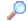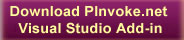SearchDirectory

Desktop Functions:

Smart Device Functions:GlossaryShow Recent ChangesSubscribe (RSS)Misc. Pages
SendInput (user32)

Summary

#### C# Signature:

[DllImport("user32.dll")]
static extern uint SendInput(uint nInputs, INPUT [] pInputs, int cbSize);

#### VB.NET Signature:

Private Declare Function SendInput Lib "user32.dll" (ByVal cInputs As Integer, ByRef pInputs As INPUT, ByVal cbSize As Integer) As Integer

#### C# User-Defined Types:

[StructLayout(LayoutKind.Sequential)]
struct MOUSEINPUT
{
int dx;
int dy;
int mouseData;
int dwFlags;
int time;
IntPtr dwExtraInfo;
}

[StructLayout(LayoutKind.Sequential)]
struct KEYBDINPUT
{
short wVk;
short wScan;
int dwFlags;
int time;
IntPtr dwExtraInfo;
}

[StructLayout(LayoutKind.Sequential)]
struct HARDWAREINPUT
{
int uMsg;
short wParamL;
short wParamH;
}

[StructLayout(LayoutKind.Explicit)]
struct INPUT
{
[FieldOffset(0)] int type;
[FieldOffset(4)] MOUSEINPUT mi;
[FieldOffset(4)] KEYBDINPUT ki;
[FieldOffset(4)] HARDWAREINPUT hi;
}

#### VB.NET User-Defined Types:

<StructLayout(LayoutKind.Explicit)> _
Private Structure INPUT
<FieldOffset(0)> Dim dwType As Integer
<FieldOffset(4)> Dim mouseInput As mouseInput
<FieldOffset(4)> Dim keyboardInput As KEYBDINPUT
<FieldOffset(4)> Dim hardwareInput As hardwareInput
End Structure

<StructLayout(LayoutKind.Explicit)> _
Private Structure KEYBDINPUT
<FieldOffset(0)> Public wVk As Short
<FieldOffset(2)> Public wScan As Short
<FieldOffset(4)> Public dwFlags As Integer
<FieldOffset(8)> Public time As Integer
<FieldOffset(12)> Public dwExtraInfo As IntPtr
End Structure

<StructLayout(LayoutKind.Explicit)> _
Private Structure HARDWAREINPUT
<FieldOffset(0)> Public uMsg As Integer
<FieldOffset(4)> Public wParamL As Short
<FieldOffset(6)> Public wParamH As Short
End Structure

<StructLayout(LayoutKind.Explicit)> _
Private Structure MOUSEINPUT
<FieldOffset(0)> Public dx As Integer
<FieldOffset(4)> Public dy As Integer
<FieldOffset(8)> Public mouseData As Integer
<FieldOffset(12)> Public dwFlags As Integer
<FieldOffset(16)> Public time As Integer
<FieldOffset(20)> Public dwExtraInfo As IntPtr
End Structure

None.

#### Sample Code:

// It might be better to write this code as a single call to SendInput passing
// all the key downs and keys ups in one array.  If you do this, SendInput
// guarantees that no other keys get inbetween the whole sequence.  As is stands,
// a "real" key transition could in theory sneak in the middle - and if it did
// it would inherit the states of shift, control, etc this program had set up.

class Test
{
public enum VK : ushort
{
SHIFT        = 0x10,
CONTROL          = 0x11,
ESCAPE           = 0x1B,
BACK         = 0x08,
TAB          = 0x09,
RETURN           = 0x0D,
PRIOR        = 0x21,
NEXT         = 0x22,
END          = 0x23,
HOME         = 0x24,
LEFT         = 0x25,
UP           = 0x26,
RIGHT        = 0x27,
DOWN         = 0x28,
SELECT           = 0x29,
PRINT        = 0x2A,
EXECUTE          = 0x2B,
SNAPSHOT         = 0x2C,
INSERT           = 0x2D,
DELETE           = 0x2E,
HELP         = 0x2F,
MULTIPLY         = 0x6A,
SEPARATOR        = 0x6C,
SUBTRACT         = 0x6D,
DECIMAL          = 0x6E,
DIVIDE           = 0x6F,
F1           = 0x70,
F2           = 0x71,
F3           = 0x72,
F4           = 0x73,
F5           = 0x74,
F6           = 0x75,
F7           = 0x76,
F8           = 0x77,
F9           = 0x78,
F10          = 0x79,
F11          = 0x7A,
F12          = 0x7B,
OEM_1        = 0xBA,   // ',:' for US
OEM_PLUS         = 0xBB,   // '+' any country
OEM_COMMA        = 0xBC,   // ',' any country
OEM_MINUS        = 0xBD,   // '-' any country
OEM_PERIOD       = 0xBE,   // '.' any country
OEM_2        = 0xBF,   // '/?' for US
OEM_3        = 0xC0,   // '`~' for US
MEDIA_NEXT_TRACK     = 0xB0,
MEDIA_PREV_TRACK     = 0xB1,
MEDIA_STOP           = 0xB2,
MEDIA_PLAY_PAUSE     = 0xB3,
LWIN       =0x5B,
RWIN       =0x5C
}

public struct KEYBDINPUT
{
<FieldOffset(0)>public ushort wVk;
<FieldOffset(4)>public ushort wScan;
<FieldOffset(8)>public uint dwFlags;
<FieldOffset(12)>public long time;
<FieldOffset(16)>public uint dwExtraInfo;
};
[StructLayout(LayoutKind.Explicit,Size=28)]
public struct INPUT
{
<FieldOffset(0)> public uint type;
<FieldOffset(4)> public KEYBDINPUT ki;
};

void Test()
{
INPUT structInput;
structInput = new INPUT();
structInput.type = Win32Consts.INPUT_KEYBOARD;

// Key down shift, ctrl, and/or alt
structInput.ki.wScan = 0;
structInput.ki.time = 0;
structInput.ki.dwFlags = 0;
structInput.ki.dwExtraInfo = Win32.GetMessageExtraInfo();
if (Shift)
{
structInput.ki.wVk = (ushort)VK.SHIFT;
intReturn = Win32.SendInput(1, ref structInput, (UInt32)sizeof(INPUT));
}
if (Ctrl)
{
structInput.ki.wVk = (ushort)VK.CONTROL;
intReturn = Win32.SendInput(1, ref structInput, (UInt32)sizeof(INPUT));
}
if (Alt)
{
intReturn = Win32.SendInput(1, ref structInput, (UInt32)sizeof(INPUT));
}
if (Win)
{
structInput.ki.wVk = (ushort)VK.LWIN;
intReturn = Win32.SendInput(1, ref structInput, (UInt32)sizeof(INPUT));
}
// Key down the actual key-code
structInput.ki.wVk = vk;
intReturn = Win32.SendInput(1, ref structInput, (UInt32)sizeof(INPUT));
// Key up the actual key-code
structInput.ki.dwFlags = Win32Consts.KEYEVENTF_KEYUP;
intReturn = Win32.SendInput(1, ref structInput, (UInt32)sizeof(INPUT));
// Key up shift, ctrl, and/or alt
if (Shift)
{
structInput.ki.wVk = (ushort)VK.SHIFT;
intReturn = Win32.SendInput(1, ref structInput, (UInt32)sizeof(INPUT));
}
if (Ctrl)
{
structInput.ki.wVk = (ushort)VK.CONTROL;
intReturn = Win32.SendInput(1, ref structInput, (UInt32)sizeof(INPUT));
}
if (Alt)
{
intReturn = Win32.SendInput(1, ref structInput, (UInt32)sizeof(INPUT));
}
if (Win)
{
structInput.ki.wVk = (ushort)VK.LWIN;
intReturn = Win32.SendInput(1, ref structInput, (UInt32)sizeof(INPUT));
}
}
}

#### VB.NET Sample Code:

Private Sub SendInputStream()
' Synthesize the user clicking the right mouse button.
Dim inputevents As New Input              ' holds information about each event
Dim mouseevent As MOUSEINPUT              ' temporarily hold mouse input info

' Load the information needed to synthesize pressing the right mouse button.
inputevents.mouseInput.dx = 0              ' no horizontal movement
inputevents.mouseInput.dy = 0              ' no vertical movement
inputevents.mouseInput.mouseData = 0              ' not needed
inputevents.mouseInput.dwFlags = MOUSEEVENTF_RIGHTDOWN              ' right button down
inputevents.mouseInput.time = 0              ' use the default
inputevents.mouseInput.dwExtraInfo = GetMessageExtraInfo()              ' not needed
' Copy the structure into the input array's buffer.
inputevents.dwType = INPUT_MOUSE              ' mouse input
' Now that all the information for the four input events has been placed
' into the array, finally send it into the input stream.
SendInput(1, inputevents, Marshal.SizeOf(inputevents))               ' place the events into the stream

' Do the same as above, but for releasing the right mouse button.
inputevents.mouseInput.dx = 0              ' no horizontal movement
inputevents.mouseInput.dy = 0              ' no vertical movement
inputevents.mouseInput.mouseData = 0              ' not needed
inputevents.mouseInput.dwFlags = MOUSEEVENTF_RIGHTUP              ' right button up
inputevents.mouseInput.time = 0              ' use the default
inputevents.mouseInput.dwExtraInfo = GetMessageExtraInfo()              ' not needed
' Copy the structure into the input array's buffer.
inputevents.dwType = INPUT_MOUSE              ' mouse input
SendInput(1, inputevents, Marshal.SizeOf(inputevents))               ' place the events into the stream
End Sub

#### Alternative Managed API:

Do you know one? Please contribute it!

Documentation
SendInput on MSDN

Do you have...

• helpful tips or sample code to share for using this API in managed code?
• corrections to the existing content?
• variations of the signature you want to share?
• additional languages you want to include?

Select "Edit This Page" on the right hand toolbar and edit it! Or add new pages containing supporting types needed for this API (structures, delegates, and more).Access PInvoke.net directly from VS:Terms of UseFind ReferencesShow Printable VersionRevisions Show changes